# Why You Should Never Use Pixelation To Hide Sensitive Text

Undoubtedly you have all seen photographs of people on TV and online who have been blurred to hide faces. For example, here's one of Bill Gates: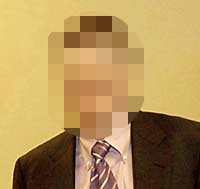For the most part this is all fine with peoples' faces as there isn't a convenient way to reverse the blur back into a photo so detailed that you can recognise the photo. So that's good if that is what you intended. However, many people also resort to blurring sensitivenumbers andtext. I'll illustrate why that is a BAD idea.

Suppose someone posted a photo of their check or credit card online for whatever awful reason (proving to Digg that I earned a million dollars, showing something funny about a check, comparing the size of something to a credit card, etc.), blurring out the image with the far-too-common mosaic effect to hide the numbers: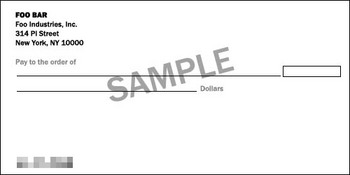Seem secure because nobody can read the numbers anymore? WRONG. Here's a way to attack this scheme:

Step 1. Get a blank check image.
There are two ways of doing this. You can either Photoshop out the numbers in your existing image, or in the case of credit cards, you can get an account with the same organization and take a photo of your own card from the same angle, and match the white balancing and contrast levels. Then, use your own high resolution photo to photoshop out your numbers.
This is easy in these example images, of course: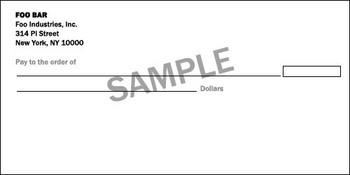Step 2. Iterate.
Use a script to iterate through all the possible account numbers and generate a check for each, blocking out the various sections of digits as sections. For example, for a VISA card, the digits are grouped by 4, so you can do each section individually, thus requiring only 4*10000 = 40000 images to generate, which is easy with a script.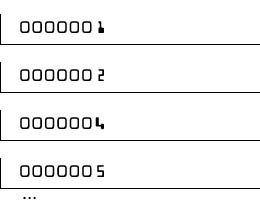Step 3. Blur each image in an identical manner to the original image.
Identify the exact size and offset, in pixels, of the mosaic tiles used to blur the original image (easy), and then do the same to each of your blurred images. In this case, we see that the blurred image we have 8x8 pixel mosaic units, and the offset is determined by counting from the top of the image (not shown):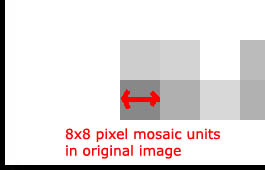Now we iterate through all the images, blurring them in the same way as the original image and obtain something like this: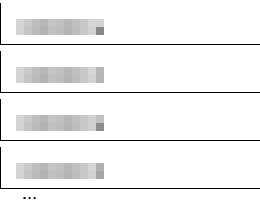Step 4. Identify the mozaic brightness vector of each blurred image.
What does this mean? Well, let's take the mozaic version of 0000001 (zoomed in):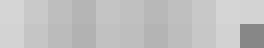... and identify the brightness level (0-255) of each mozaic region, indexing them in some consistent fashion as a=[a_1,a_2...,a_n]: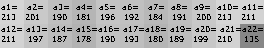In this case, the account number 0000001 creates mozaic brightness vector a(0000001)=[213,201,190,...]. We find the mozaic brightness vector for every account number in a similar fashing using a script to blur each image and read off the brightnesses. Let a(x) be the function of the account number x. a(x)_i denotes the ith vector value of the mozaic brightness vector a obtained from account number x. Above, a(0000001)_1 = 213.

We now do the same for the original check image we found online or wherever, obtaining a vector we hereby call z=[z_1,z_2,...z_n]: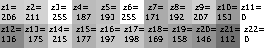Step 4. Find the one with the closest distance to the original image.
Identify the mozaic brigtness of the original image, call it z=[z_1,z_2,...z_n], and then simply compute the distance of each account number's (denote by x) mozaic brightness vector (normalizing each first):

d(x)=sqrt((a(x)_0/N(a(x)) - z_0/N(z))^2 + (a(x)_1/N(a(x)) - z_1/N(z))^2 + ...)

where N(a(x)) and N(z) are the normalization constants given by

N(a(x)) = (a(x)_0^2 + a(x)_1 ^2 + ...)^2
N(z) = (z_0^2 + z_1 ^2 + ...)^2

Now, we then simply find the lowest d(x). For credit cards, only a small fraction of possible numbers validate to hypothetically possible credit card numbers, so it's an easy check as well.

In the above case, we compute, for example,

N(z) = sqrt(206^2+211^2+...) = 844.78459
N(a(0000001)) = 907.47837
N(a(0000002)) = 909.20647
...

and then proceed to calculate the distances:

d(0000001) = 1.9363
d(0000002) = 1.9373
...
d(1124587) = 0.12566
d(1124588) = 0.00000
...

Might the account number just be 1124588?

"But you used your own crafted easy-to-decipher image!"
In the real world we have photos, not fictitious checks made in Photoshop. We have distortions of the text because of the camera angle, imperfect alignment, and so on. But that doesn't stop a human from determining exactly what these distortions are and creating a script to apply them! Either way, the lowest few distances determined can be considered as candidates, and especially in the world of credit cards, where numbers are nicely chunked out in groups of 4, and only 1 in 10 numbers is actually a valid number, it makes it easy to select from your top few lowest distances, which the most likely candidates are.

One important thing that one would need to do in order to implement this on real photos is to improve the distance algorithm. For example, one can rewrite the distance formula above to normalize the standard deviations in addition to the means to improve performance. One can also do the RGB or HSV values independently for each mozaic region, and one can also use scripting to distort the text by a few pixels in each direction and compare as well (which still leaves you with a feasible number of comparisons on a fast PC). One can also employ algorithms similar to existing nearest-shape algorithms to help improve the reliability of this on real photos.

So yes, I used an image against itself and designed it to work here. But the algorithem can surely be improved to work on real stuff. I don't have the time nor desire to improve this any further, though, because I'm not the one after your information. But one thing is for sure: it's a very easy situation to fix. Don't use simple mosaics to blur your image. All you do is reduce the amount of information from an image containing only log(10^N)/log(2) effective bits of account data. When you distribute such images, you want toeliminate personal information, not obscure it by reducing the amount of visual information in the image.

Think about creating a 100x100 graphic on the screen. now lets say i just averaged out the entire graphic and replaced every pixel with the whole average (i.e. turn it into a single pixel "mosaic"). You have just created a function that starts with 256^(10000) possibilities and hashes it to 256 possibilities. There is obviously no way with the resulting 8 bits of information you can possibly reverse it to the original image. However, if you know that the original image was one of 10 possibilities, you can easily have success at determining which of the original images was used from just knowing the resulting 8-bit number.

Analogy to a dictionary attack
Most UNIX/Linux system administrators know that /etc/passwd or /etc/shadow store passwords encrypted using one-way encryption such as Salt or MD5. This is reasonably secure since nobody will ever be able to decrypt the password from looking at its ciphertext. Authentication occurs by performing the same one-way encryption on the password entered by the user logging in, and comparing that result to the stored one-way result. If the two match, the user has successfully authenticated.

It is well known that the one-way encryption scheme is easily broken when the user picks a dictionary word as their password. All an attacker would have to then do is encipher the entire English dictionary and compare the ciphertext of each word to the ciphertext stored in /etc/passwd and pick up the correct word as the password. As such, users are commonly advised to pick more complex passwords that are not words. The dictionary attack can be illustrated like this: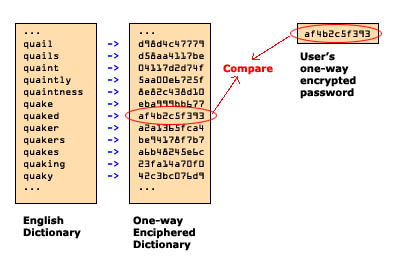The similary to the dictionary attack on the blured image attack lies in the fact that blurring an image is a one-way encryption scheme. You are converting the image you have into another image designed to be unreadable. However, since account numbers only typically go up to the millions, we can assemble a "dictionary" of possible account numbers - that is, all the numbers from 0000001 to 9999999, for example, use an automated image processor to photoshop each of those numbers onto a photo of a blank check, and blur each image. At that point, one can simply compare the blurred pixels to see whatmost closely matches the original blurred photo we have.

Solution
The solution is simple: Don't blur your images! Instead, just color over them: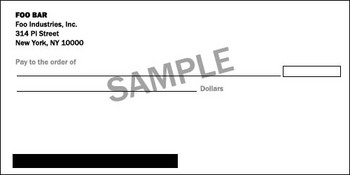Remember, you want to leave your visitors with NO information, not blurred information.